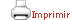Teaching plan for the course unit

 CloseGeneral information

Course unit name: Tensor Networks

Course unit code: 574645

Coordinator: Bruno Julia Diaz

Department: Department of Quantum Physics and Astrophysics

Credits: 3

Single program: S

 Estimated learning time Total number of hours 75

 Face-to-face and/or online activities 26
 -  Lecture Face-to-face and online 20 -  Lecture with practical component Face-to-face and online 6
 Independent learning 49

 Recommendations

 Students are expected to have a solid background in quantum mechanics and statistical mechanics and master one programming language.

 Competences to be gained during study

 Ability to understand Tensor Network approaches, use the most common tensor network packages and produce their own codes.

 Learning objectives
 Referring to knowledge To develop a broad and unified perspective on tensor networks.  To become familiar with the basic tensor network structures like Matrix product states, Tree Tensor networks and Projected entangled pair states.  To understand the fundamentals numerical algorithms used in the context of tensor networks.

 Teaching blocks

1. Generalities

*

1. An introduction to the tensor network formalism and graphical notation,

2. Introduction to the tensor network zoo, MPS, PEPS, TTN, MERAs ...

2. Analytical foundation of tensor networks

*

1. Matrix product states and their representation

2. Canonical form and injectivity

3. Entanglement  in many-body quantum systems

4. The geometry of tensor networks

3. Tensor networks approaches to statistical mechanics

*

1. Encoding partition functions

2. Calculating entropies

4. Back to quantum systems

*

1. Transfer matrix and the quantum Hamiltonian

2. The renormalization group

3. DMRG as a RG algorithm

5. Numerical simulation the existing software

*

1. An overview of the steps in numerical algorithms

2. Tensor contractions, their cost and strategies

3. Overview of the main software packages

6. Numerical simulations building new algorithms

*

1. Guiding principles

2. Software libraries helping designing new algorithms

7. Symmetries in tensor Networks

*

1. The physical relevance of symmetry

2. Symmetries in tensor networks

3. Implementing symmetries in practice

 Official assessment of learning outcomes

 Continuous assessment, based on working on a final project. The project will contain several sections that will be evaluated during the course.  Some sections will be common to all projects (the basic ones).  Exercises will be also provided, and their solution will contribute to the final mark.

Book

Tensor Network Contractions Methods and Applications to Quantum Many-Body Systems Authors: Ran, S.-J., Tirrito, E., Peng, C., Chen, X., Tagliacozzo, L., Su, G., Lewenstein, M.  Springer Lecture notes in PhysicsVideo, DVD and film

Topological Matter School. TMS18.L25. Frank Pollmann. Tensor Networks and Matrix Product States (I).; 2018. Accessed January 25, 2021.ICTP Condensed Matter and Statistical Physics. Norbert Schuch Tensor Networks - Lecture 1.; 2017. Accessed January 25, 2021.Cornell Laboratory of Atomic and Solid State Physics. Garnet Chan “Matrix Product States, DMRG, and Tensor Networks” (Part 1 of 2).; 2015. Accessed January 25, 2021.ICAM - I2CAM. Tensor Network States & Entanglement Renormalization I - Verstraete.; 2016. Accessed January 25, 2021.Article

J. C. Bridgeman and C. T. Chubb, Hand-Waving and Interpretive Dance: An Introductory Course on Tensor Networks, J. Phys. A: Math. Theor. 50, 223001 (2017).

Haegeman and F. Verstraete, Diagonalizing Transfer Matrices and Matrix Product Operators: A Medley of Exact and Computational Methods, ArXiv:1611.08519 [Cond-Mat, Physics:Math-Ph, Physics:Quant-Ph] (2016).

R. Orus, A Practical Introduction to Tensor Networks: Matrix Product States and Projected Entangled Pair States, Annals of Physics 349, 117 (2014).